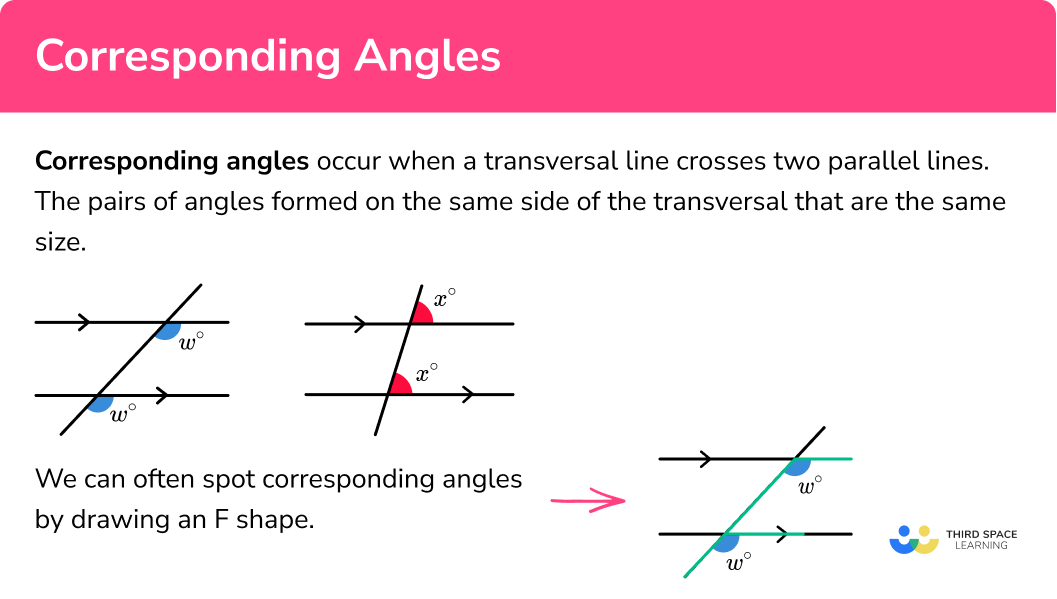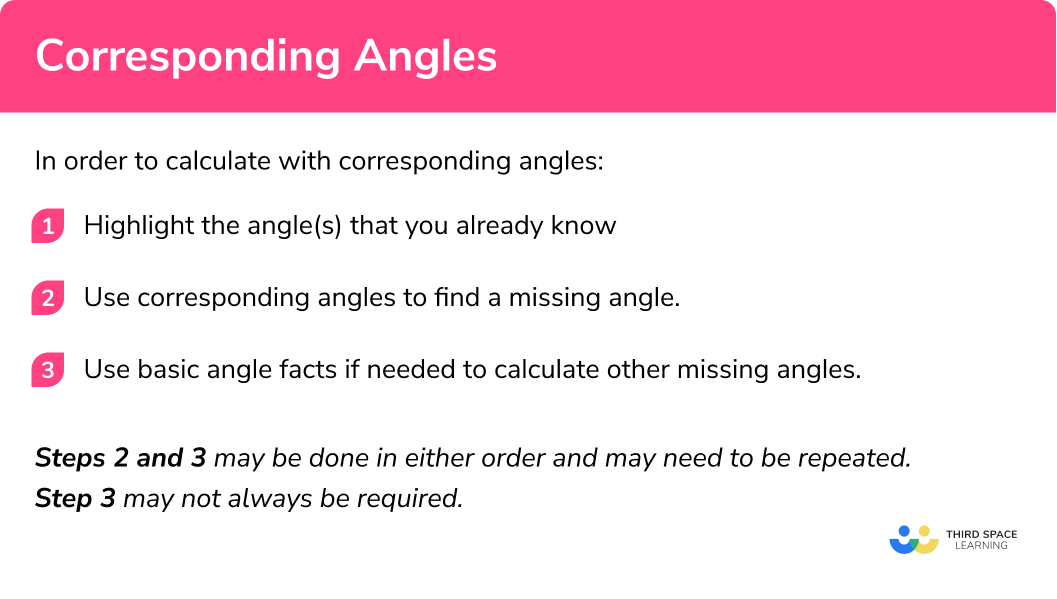# Corresponding Angles

Here we will learn about corresponding angles including how to recognise when angles are corresponding and apply this to solve problems.

There are also angles in parallel lines worksheets based on Edexcel, AQA and OCR exam questions, along with further guidance on where to go next if you’re still stuck.

## What are corresponding angles?

Corresponding angles are angles that occur on the same side of the transversal line and are equal in size. They are either both obtuse or both acute.

Corresponding angles are equal

We can often spot corresponding angles by drawing an F shape.

### What are corresponding angles?## How to calculate with corresponding angles

In order to calculate with corresponding angles:

1. Highlight the angle(s) that you already know.
2. Use corresponding angles to find a missing angle.
3. Use a basic angle fact to calculate the missing angle.

### How to calculate with corresponding angles## Corresponding angles examples

### Example 1: corresponding angles

1. Highlight the angle(s) that you already know.

2 Use corresponding angles to find a missing angle.

Here we can label the corresponding angle on the diagram as 75°.

3Use a basic angle fact to calculate the missing angle.

Here θ is on a straight line with 75°

\begin{aligned} \theta&=180^{\circ}-75^{\circ} \\ \theta&=105^{\circ} \end{aligned}

### Example 2: corresponding angles

We can identify two new angles of 63° and 56° by using corresponding angles.

As the sum of angles in a triangle is 180°

\begin{aligned} \theta&=180^{\circ}-\left(63^{\circ}+56^{\circ}\right) \\ \theta&=61^{\circ} \end{aligned}

### Example 3: corresponding angles with algebra

Here we need to find the value for x to find the value of θ.

The relative position of the two angles 4x -14 and 3x+7 make them corresponding angles. This allows us to find the value of x as the two angles are equal:

\begin{aligned} 4x-14&=3 x+7 \\ 4x&=3x+21 \\ x&=21^{\circ} \end{aligned}

As x = 21°, we have the two angles 4x – 14 = 3x + 7 = 70°.

Here we can combine the fact that we have an isosceles triangle and the sum of angles on a straight line is 180°

As the triangle is isosceles with the base of the triangle on one parallel line, the angles either side of the top vertex of the triangle are symmetrical (this can also be calculated using the alternate angle rule).
These angles form a straight line, and as angles on a straight line add to 180 we can therefore find θ:

\begin{aligned} \theta&=180-(70+70) \\ \theta&=40^{\circ} \end{aligned}

### Common misconceptions

• Mixing up angle facts

There are a lot of angle facts and it is easy to mistake alternate angles with corresponding angles. To prevent this from occurring, think about corresponding angles as being underneath the F shape.

• Using a protractor to measure an angle

Most diagrams are not to scale and so using a protractor will not result in a correct answer.

Corresponding angles is part of our series of lessons to support revision on angles in parallel lines. You may find it helpful to start with the main angles in parallel lines lesson for a summary of what to expect, or use the step by step guides below for further detail on individual topics. Other lessons in this series include:

### Practice corresponding angles questions

1. Calculate the size of angle \theta\theta=96^{\circ}\theta=84^{\circ}\theta=264^{\circ}\theta=74^{\circ}Using corresponding angles, we can see the angle 96^{\circ} . We can then use angles on a straight line: \theta=180-96=84^{\circ} .2. Calculate the value of \theta .\theta=72^{\circ}\theta=36^{\circ}\theta=108^{\circ}\theta=144^{\circ}Using corresponding angles, we can see the angle \theta=72^{\circ} . We can then use angles on a straight line: \theta=180-72=108^{\circ}3. Work out the size of angle \theta\theta=60^{\circ}\theta=58^{\circ}\theta=62^{\circ}\theta=122^{\circ}We start by calculating the missing angle in the triangle: 180-(62+60)=58 . We can then use corresponding angles to see that \theta=58^{\circ} .4. Calculate the value for angle \theta\theta=106^{\circ}\theta=95^{\circ}\theta=115^{\circ}\theta=65^{\circ}Using corresponding angles, we can see that \theta is corresponding to 106+11 therefore \theta=106+11=115^{\circ}5. Calculate the size of angle \theta\theta=12^{\circ}\theta=97^{\circ}\theta=109^{\circ}\theta=85^{\circ}Using corresponding angles, we can see the angle 97^{\circ} . Since vertically opposite angles are equal, the angle opposite is also 97^{\circ} . Using corresponding angles we can also see the angle 12^{\circ} . Then \theta=97-12=85^{\circ} .6. By calculating the value for x , work out the size of each of the corresponding angles labelled in the diagram.45^{\circ} \text{ and } 45^{\circ}45^{\circ} \text{ and } 135^{\circ}30^{\circ} \text{ and } 20^{\circ}50^{\circ} \text{ and } 50^{\circ}3x and 5x-30 are corresponding angles and so are equal. Therefore we can write 3x=5x-30 .
We can then solve this:
\begin{aligned} 3x&=5x-30\\ 0&=2x-30\\ 30&=2x\\ 15&=x \end{aligned}
\begin{array}{l} 3\times 15 = 45^{\circ}\\ 5\times 15-30 = 45^{\circ} \end{array}

### Corresponding Angles GCSE questions

1.(a) Work out the size of angle m. Give reasons for your answer.

(b) Work out the size of angle n.

(4 marks)

(a) m and 74 ^{\circ} are corresponding

(1)

m=74^{\circ}

(1)

(b) Corresponding angles are equal:(1)

Angles on a straight line add to 180 so,
180-74=106^{\circ}

(1)

2.(a) Work out the size of angle a.

(b) Work out the size of angle b.

(3 marks)

(a) a=37^{\circ} as corresponding angles are equal

(1)

(b) 98^{\circ} is corresponding with b+37^{\circ}
so b+37=98

(1)

b=61^{\circ}

(1)

3. Work out the value of x .(3 marks)

Opposite angles equal(1)

2x+9=4x-3 as corresponding angles are equal

(1)

\begin{aligned} 2x+9&=4x-3\\ 9&=2x-3\\ 12&=2x\\ 6&=x \end{aligned}

(1)

## Learning checklist

You have now learned how to:

• Apply the properties of angles at a point, angles at a point on a straight line, vertically opposite angles
• Understand and use the relationship between parallel lines and alternate and corresponding angles

## Still stuck?

Prepare your KS4 students for maths GCSEs success with Third Space Learning. Weekly online one to one GCSE maths revision lessons delivered by expert maths tutors.

Find out more about our GCSE maths tuition programme.